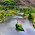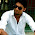## Counting Sort Algorithm with Example

After a long interval of time I am writing a post on ALGORITHMS. After writing posts on Heap Sort, Merge Sort and Insertion Sort, I decided to write on Counting Sort.

Counting sort is an algorithm for sorting a collection of objects according to keys that are small integers; that is, it is an integer sorting algorithm. It is a linear time sorting algorithm used to sort items when they belong to a fixed and finite set.
The algorithm proceeds by defining an ordering relation between the items from which the set to be sorted is derived (for a set of integers, this relation is trivial).Let the set to be sorted be called A. Then, an auxiliary array with size equal to the number of items in the superset is defined, say B. For each element in A, say e, the algorithm stores the number of items in A smaller than or equal to e in B(e). If the sorted set is to be stored in an array C, then for each e in A, taken in reverse order, C[B[e]] = e. After each such step, the value of B(e) is decremented.

# Algorithm :

In n the code for Counting sort, we are given array A[1 . . n] of length n. We required two more arrays, the array B[1 . . n] holds the sorted output and the array c[1 . . k] provides temporary working storage.

## Example :

Each line below shows the step by step operation of counting sort.

Suppose that the values to be sorted are between 0 and k, where k is some (small) integer. Assume the values are in the array A[1..n].

1. Use an array C[0..k] to count how many times each key occurs in A. This requires Θ(n) time.
2. Calculate cumulative totals in C. The time is Θ(k). These numbers tell us, for example, that the three occurrences of 3 should be in places 5,6,7 of the ﬁnal array.
3. Copy data into the target array B. The time is Θ(n)

for i = n downto 1
B[C[A[i]]] ←− A[i]
C[A[i]] ←− C[A[i]] − 1

4. Assuming k = O(n), the total time is O(n)—better than any comparison sort.

Note that the counting sort is stable: it preserves the ordering of elements that have the same key. (Previously seen sorting algorithms do not have this property, but some do
have stable versions.)

 A 3 6 4 1 3 4 1 4 C 2 0 2 3 0 1

 2.C 2 2 4 7 7 8

 B 4 C 2 2 4 6 7 8

 B 1 4 C 1 2 4 6 7 8

 B 1 4 4 C 2 2 4 5 7 8

 B 1 1 3 3 4 4 4 6

## Analysis :

1. The loop of lines 1-2   takes O(k) time
2. The loop of lines 3-4   takes O(n) time
3. The loop of lines 6-7   takes O(k) time
4. The loop of lines 9-11 takes O(n) time

Therefore, the overall time of the counting sort is
O(k) + O(n) + O(k) + O(n) = O(k + n)

In practice, we usually use counting sort algorithm when have k = O(n), in which case running time is O(n).

The Counting sort is a stable sort i.e., multiple keys with the same value are placed in the sorted array in the same order that they appear in the input array.

NOTE :

If line 9 in the Algorithm is changed to : for j = 1 to n, Then the stability doesn't holds true.

## Source Code (Java) :

1.Nice description.. I folllow ur post regularly and they are very informative.

2.3.Didn't know about this sorting method before,Thanks for sharing. while programming in Java you don't get to writing your own sorting code instead you can use Collections.sort for sorting ArrayList in java or any other collection.

4.Tnks
It is Easy to understand

5.Nice explanation, while showing the phases the count array that is 'C' the range start from "0 to K" & in your example the value of "K" is "6". But you have shown that "C" array starts with "1 to 6" which I think should be "0 to 6".

Kindly explain...

6.more details plzzz ?

7.how C is 2 0 2 3 0 1
it should be according to algorithm
2 1 3 2
8.9.